# Atomic Energy Levels

Atoms constitute the building blocks of all materials in existence. In these atoms, there is a central portion called nucleus (N in Figure 1) which consists of protons and neutrons, around which revolves the particles called electrons. Next, it is to be noted that all the electrons constituting the considered material do not revolve along the same path. However this even does not mean that their revolutionary paths can be random. That is, each electron of a particular atom has its own dedicated path, called orbit, along which it circles around the central nucleus. It is these orbits which are referred to as energy levels of an atom.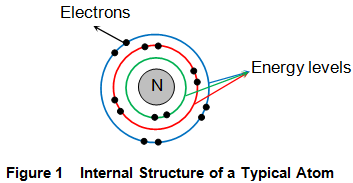This is because, each of them possess a dedicated amount of energy which is expressed in terms of an integral multiple of the equation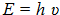Where h is the Planck’s constant and υ is the frequency.

Figure 2 shows the finite energy possessed by different energy states (and thereby all the electrons present in them) in electron volts (eV). From the figure, it can be seen that the energy of the electrons increases as one moves away from the center of the atom. For example, an electron in the first energy state (E1) has an energy of -13.6 eV, that in the second (E2) possess an energy of -3.4 eV and so on. Continuing so, one may reach a level at which the energy becomes 0 eV i.e. the energy level E.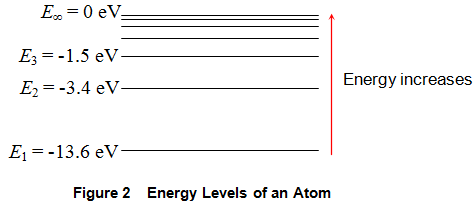Now assume that we are supplying external energy (might be in any way including that of light) to the material. This energy supplied will be absorbed by the electrons present in the atoms constituting the material. However the electrons are not let to absorb any amount of energy as they wish-for. This is because, if an electron absorbs some energy, then its net energy changes. This inturn means that the electron can no longer stay in its original energy level. Say for example, an electron in the energy state E1 absorbs 4 eV of energy. On doing so, the net energy of the electron would increase to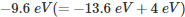due to which it can no longer stay in the energy level E1 which has its energy as -13.6 eV. Moreover it cannot see any other level which has an energy equivalent to what it has. This makes it lose its track!

On the other hand, if this electron absorbs energy of 10.2 eV, then its increased energy would be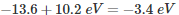This is nothing but the energy possessed by the level E2, meaning which the electron which was formerly in E1 is now in the energy level E2. In other words, we say that this electron has made a transition from the level E1 to the level E2 which inturn leads to an excited atom. However the electron cannot stay in this unstable state for long. It will soon return to its original state by making a transition from the level E2 to the level E1. But an important point to be noted here is the fact that while doing so, the electron emits an energy of 10.2 eV (which is same as that of the absorbed) in the form of electromagnetic waves.

From the discussion presented, it is evident that the electrons are permitted to absorb (or equivalently emit) only quantized amounts of energy. The amount of this energy is nothing but the difference in the energies of the levels among which the transition occurs. Next, from Figure 2, it is seen that this difference between the energy states goes on decreasing as one moves away from E1 i.e. …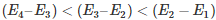This means that the electrons in the outermost shells require less amount of energy to get excited than those present in the innermost shells. This is in accordance with the well known fact that the electrons present near the nucleus are strongly bonded to the atoms rather than the ones which are present away from it.
Although we have explained the process of excitation, the same mode of argument holds good even for the case of liberation.This is because, we can assume that the electron when gets excited to the energy level with an energy of 0 eV (E), it would be completely free from the attractive force of the atom’s nucleus. It is these free electrons which contribute for conduction in the case of materials like metals.

Want To Learn Faster? 🎓
Get electrical articles delivered to your inbox every week.
No credit card required—it’s 100% free.